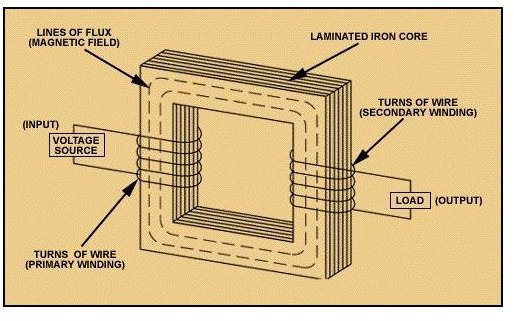# Transformers – Transfer Power (as if) by Magic

## Introduction

You must have watched magical shows personally or on television and one of the favourite tricks of a magician is to transfer an object or a person from one box to another without any actual transfer of matter. Well this may seem a very crude analogy but it would help you to visualize what goes on inside a transformer. So let us get down to some serious study now.

## What is a Transformer?

A transformer can be defined as an electrical device in which there are two circuits, and electric power is transferred between these two circuits without any actual contact, simply by mutual induction. A simple transformer consists of a laminated steel core around which the two circuit wires are wound. The circuit from which energy is fed into the transformer is known as the primary circuit, while the circuit from which energy is drawn from the transformer is known as secondary circuit, or the "load". The image in figure 1 below shows such a transformer.

## Figure 1: Schematic of a Transformer## Why a Transformer?

Nothing exists without purpose and there should be a reason why electricity is fed from the primary circuit into the secondary circuit through a transformer and not directly. The reason is that a transformer can be used to vary the voltage (and the current) from the primary circuit to the secondary circuit. This variation could be either way, which means to say that it can either be increased or decreased. There are numerous occasions where this transformation of voltage and current is required, some of which are given below

• To match the source of power and source of consumption when they do not have same voltage ratings
• When electricity is transmitted over long distances, it needs to have high voltage to avoid losses

We know that the principle of conservation of energy states that energy cannot be created or destroyed but only changed in form. When this principle is applied to a transformer, effectively it means to say that whatever energy is fed into the input or primary circuit should come out of the secondary circuit (minus any losses within the transformer). Let us do a bit of mathematical analysis of this phenomenon.

Power in an electrical circuit is given by the equation P = V*I where P, V and I stand for power, voltage and current respectively. So, for a given amount power, the voltage and current are inversely proportional to each other. This means that if the voltage is reduced, the current increases and vice-versa. This is what happens inside the transformer (we will study this in more detail in the forthcoming articles of this series) and the voltage is either increased (step up transformer) or decreased (step down transformer) from the primary to the secondary circuit.

## Application to Power Transmission

The electricity generated at power stations needs to be transmitted over long distances to towns and cities through transmission cables. So it should be ensured that minimum power losses occur during this transmission otherwise much of the efforts and fuel at the power station would be going to waste. Transformers come to our rescue in this endeavour and this is how it works.

At the power station the power generated is increased in voltage using a step up transformer to the order of several hundreds of kilovolts, depending on the application. This is then transmitted to substations, which are located in proximity to the actual consumers. The substations reduce this voltage using a step down transformer to the normal usage levels, which could be 110, 220 or 440 Volts AC.

If you are wondering how this helps to reduce loss let me explain it for you. The power loss in an electric circuit due to heat is given by the equation:

Power loss = I^2*R —– (Equation 1) (Note, R is the resistance of the circuit.)

Since Power = Voltage * Current, the above equation can also be written as follows by solving for current and substituting:

Line Loss = P^2 * R/V^2 —- (Equation 2)

Since power required by a substation is fixed for a given case, as well as R is fixed for a given installation of cables, the line loss is inversely proportional to the square of the voltage from equation 2.

The value of R depends on the material used for transmission cables and their dimensions, so for a given setup of transmission cables, the power dissipated as heat can be reduced by lowering the current, which means by increasing the voltage.

There is another equation which is the power loss fraction and is given by line loss/power transmitted or

Power loss fraction = P * R/V^2 —- (Equation 3)

Hence we can also see that the fraction of power lost increases with the increase of transmitted power.

There is an upper limit to which the voltage is increased because at very high values of voltages other types of losses such as corona discharge assume significant proportions, and requires extra insulation costs which offset the advantages of less power loss, though their detailed study is beyond the scope of this article

So much for the reasons, but what is the actual mechanism through which the electric power is transferred between the two circuits? Well you need to learn something about magnetic inductance before you could fully understand this concept. In the next article we will learn something about inductance and its uses, especially in context of the workings of the transformer. Also it would be certainly helpful if you could browse though this article about magnetic circuits as well as it pertains to the same theme. Another point worth mentioning is that transformers only work with AC (Alternating Current) and we will talk about the reasons in later articles in this series.

## References

Figure 1: Schematic of a Transformer